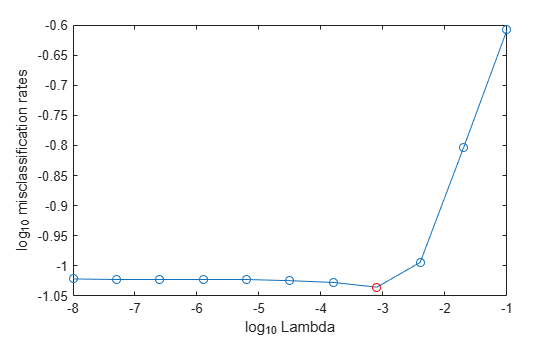# selectModels

Choose subset of multiclass ECOC models composed of binary `ClassificationLinear` learners

## Syntax

``SubMdl = selectModels(Mdl,idx)``

## Description

example

````SubMdl = selectModels(Mdl,idx)` returns a subset of trained error-correcting output codes (ECOC) models composed of `ClassificationLinear` binary models from a set of multiclass ECOC models (`Mdl`) trained using various regularization strengths. The indices (`idx`) correspond to the regularization strengths in `Mdl.BinaryLearners{1}.Lambda` and specify which models to return.`SubMdl` is returned as a `CompactClassificationECOC` model object.```

## Examples

collapse all

Choose a subset of trained ECOC models composed of linear binary learners with various regularization strengths.

Load the NLP data set.

`load nlpdata`

`X` is a sparse matrix of predictor data, and `Y` is a categorical vector of class labels.

Create a set of 11 logarithmically spaced regularization strengths from $1{0}^{-8}$ through $1{0}^{-1}$.

`Lambda = logspace(-8,-1,11);`

Create a linear classification model template that specifies optimizing the objective function using SpaRSA. Use lasso penalties with the strengths specified in `Lambda`.

```t = templateLinear('Solver','sparsa','Regularization','lasso',... 'Lambda',Lambda);```

Hold out 30% of the data for testing. Identify the test-sample indices.

```rng(1); % For reproducibility cvp = cvpartition(Y,'Holdout',0.30); idxTest = test(cvp);```

Train an ECOC model composed of linear classification models. For quicker execution time, orient the predictor data so that individual observations correspond to columns.

```X = X'; PMdl = fitcecoc(X,Y,'Learners',t,'ObservationsIn','columns','CVPartition',cvp); Mdl = PMdl.Trained{1}; numel(Mdl.BinaryLearners{1}.Lambda)```
```ans = 11 ```

`Mdl` is a `CompactClassificationECOC` model object. Because `Lambda` is an 11-dimensional vector of regularization strengths, you can think of `Mdl` as eleven trained models, each corresponding to a regularization strength.

Estimate the test-sample misclassification rates for each regularized model.

`ce = loss(Mdl,X(:,idxTest),Y(idxTest),'ObservationsIn','columns');`

Plot the misclassification rates with respect to regularization strength on the log scale.

```figure plot(log10(Lambda),log10(ce),'-o') ylabel('log_{10} misclassification rates') xlabel('log_{10} Lambda') [~,minCEIdx] = min(ce); minLambda = Lambda(minCEIdx); hold on plot(log10(minLambda),log10(ce(minCEIdx)),'ro'); hold off```Several values of `Lambda` yield similarly small classification error values. Consider choosing greater values of `Lambda` (that still yield good classification rates) because they lead to predictor variable sparsity.

Select the four models with regularization strengths that occur around the point at which the classification error starts increasing.

```idx = 7:10; MdlFinal = selectModels(Mdl,idx)```
```MdlFinal = CompactClassificationECOC ResponseName: 'Y' ClassNames: [comm dsp ecoder fixedpoint ... ] ScoreTransform: 'none' BinaryLearners: {78x1 cell} CodingMatrix: [13x78 double] Properties, Methods ```
`LambdaFinal = MdlFinal.BinaryLearners{1}.Lambda`
```LambdaFinal = 1×4 0.0002 0.0008 0.0040 0.0200 ```

`MdlFinal` is a `CompactClassificationECOC` model object. You can think of it as four models trained using the four regularization strengths in `LambdaFinal`.

## Input Arguments

collapse all

Multiclass ECOC model composed of binary linear classifiers, trained using various regularization strengths, specified as a `CompactClassificationECOC` model object.

When creating `Mdl`, you must:

• Specify `ClassificationLinear` binary learners (see `Learners`).

• Specify the same regularization strengths for each linear binary learner.

Although `Mdl` is one model object, if `numel(Mdl.BinaryLearners{1}.Lambda)` = L ≥ 2, then you can think of `Mdl` as L trained models.

Indices corresponding to regularization strengths, specified as a positive integer vector. Values of `idx` must be in the interval [1,L], where L = `numel(Mdl.BinaryLearners{1}.Lambda)`.

Data Types: `double` | `single`

## Tips

• One way to build several predictive ECOC models composed of binary linear classification models is:

1. Create a linear classification model template using `templateLinear` and specify a grid of regularization strengths using the `'``Lambda``'` name-value pair argument.

2. Hold out a portion of the data for testing.

3. Train an ECOC model using `fitcecoc`. Specify the template using the `'``Learners``'` name-value pair argument and supply the training data. `fitcecoc` returns one `CompactClassificationECOC` model object containing `ClassificationLinear` binary learners, but all binary learners contain a model for each regularization strength.

4. To determine the quality of each regularized model, pass the returned model object and the held-out data to, for example, `loss`.

5. Identify the indices (`idx`) of a satisfactory subset of regularized models, and then pass the returned model and the indices to `selectModels`. The function `selectModels` returns one `CompactClassificationECOC` model object, but it contains `numel(idx)` regularized models.

6. To predict class labels for new data, pass the data and the subset of regularized models to `predict`.

## Version History

Introduced in R2016a